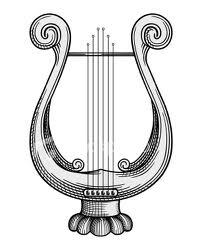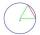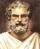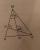# Chord MN

Chord MN of circle has distance from the center circle S 120 cm.
Angle MSN is 64°. Determine the radius of the circle.

Result

r =  141.5 cm

#### Solution:

$r = \dfrac{ 120}{ \cos(\dfrac{ 64 ^\circ }{2}) } = 141.5 \ \text{cm}$Our examples were largely sent or created by pupils and students themselves. Therefore, we would be pleased if you could send us any errors you found, spelling mistakes, or rephasing the example. Thank you!

Leave us a comment of this math problem and its solution (i.e. if it is still somewhat unclear...):Be the first to comment!Tips to related online calculators

## Next similar math problems:

1. Chord 2Point A has distance 13 cm from the center of the circle with radius r = 5 cm. Calculate the length of the chord connecting the points T1 and T2 of contact of tangents led from point A to the circle.
2. Common chordTwo circles with radius 17 cm and 20 cm are intersect at two points. Its common chord is long 27 cm. What is the distance of the centers of these circles?
3. ChordPoint on the circle is the end point of diameter and end point of chord length of radius. What angle between chord and diameter?
4. SemicircleIn the semicircle with center S and the diameter AB is constructed equilateral triangle SBC. What is the magnitude of the angle ∠SAC?
5. RT - inscribed circleIn a rectangular triangle has sides lengths> a = 30cm, b = 12.5cm. The right angle is at the vertex C. Calculate the radius of the inscribed circle.
6. Ruler and compassUse a ruler and compass to construct a triangle ABC with AB 5cm BAC 60° and ACB 45°.
7. ThalesCalculate the length of the Thales' circle described to right triangle with hypotenuse 18.4 cm.
8. FlowerbedFlowerbed has the shape of an isosceles obtuse triangle. Arm has a size 5.5 meters and an angle opposite to the base size is 94°. What is the distance from the base to opposite vertex?
9. ClimbFor horizontal distance 4.2 km road rise by 6.3 m. Calculate the road pitch in ‰ (permille, parts per thousand).
10. Center traverseIt is true that the middle traverse bisects the triangle?
11. CosineThe point (8, 6) is on the terminal side of angle θ. cos θ = ?
12. Obtuse angleThe line OH is the height of the triangle DOM, line MN is the bisector of angle DMO. obtuse angle between the lines MN and OH is four times larger than the angle DMN. What size is the angle DMO? (see attached image)
13. Right angleIf a, b and c are two sides of a triangle ABC, a right angle in A, find the value on each missing side. If b=10, c=6
14. The cable carThe cable car has a length of 3,5 kilometers and an angle of climb of 30 degrees. What is the altitude difference between Upper and Lower Station?
15. Find the 9Find the missing angle in the triangle and then name triangle. Angles are: 95, 2x+15, x+3The double ladder is 8.5m long. It is built so that its lower ends are 3.5 meters apart. How high does the upper end of the ladder reach?The double ladder shoulders should be 3 meters long. What height will the upper top of the ladder reach if the lower ends are 1.8 meters apart?